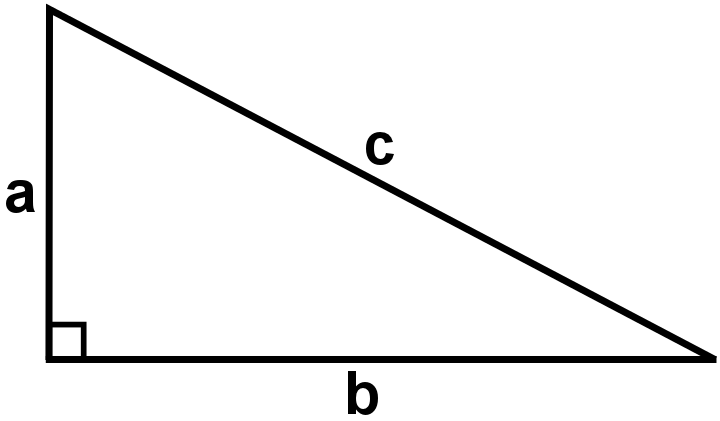### Submit a Resource

NRICH: Pythagoras Perimeters

If you know the perimeter of a right angled triangle, what can you say about the area?

If this right-angled triangle has a perimeter of 12 units, it is possible to show that the area is 366c square units.Can you find a way to prove it?
Once you’ve had a chance to think about it, click below to see a possible way to solve the problem, where the steps have been muddled up.
Can you put them in the correct order?

a) Squaring both sides: +2ab+=14424c+

b) So Area of the triangle =366c

c) a+b=12c

d) So 2ab=14424c

e) Area of the triangle =ab/2

f) By Pythagoras’ Theorem, +=

g) a+b+c=12

h) Dividing by 2ab=7212c
Printable Version

Can you adapt your method, or the method above, to prove that when the perimeter is 30 units, the area is 22515c square units?

Extension
Can you find a general expression for the area of a right angled triangle with hypotenuse c and perimeter p?

With thanks to Don Steward, whose ideas formed the basis of this problem.

Age 14 to 16

Math Topics
Algebra & Pre-Algebra, Geometry, Quadratic Equations
High School, Educator
Descriptions of PDFs

Printable version.

## Organization

NRICH (University of Cambridge)

Challenge
PDF File